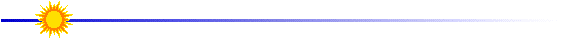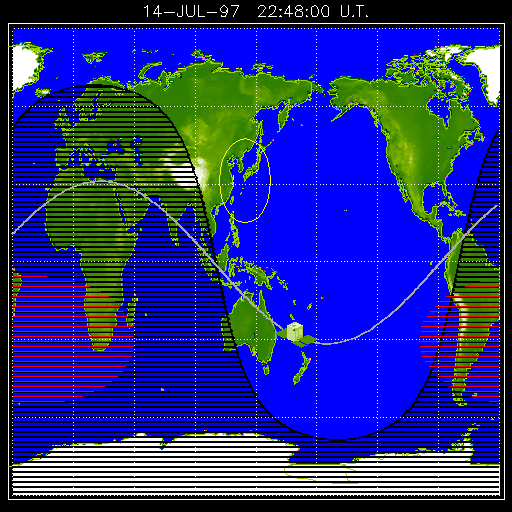# Tracking Satellites Using the Internet

Overview

Students studying physics use Internet resources to determine the orbitalperiod of satellites.  Using Newton's Universal Law of Gravity, studentsthen calculate satellite orbital velocities and altitudes. A printableversion of this activity is found here.

1. Moving Stars?
2. Internet Tracking Maps
3. Part I - Determine the orbital period of Yohkoh
4. Part II - Determine the orbital velocity of Yohkoh
5. Part III - Determine the orbiting altitude ofYohkoh
6. Monitoring a Satellite Network

Learning Outcomes

By completing this activity, the learner will:

• view real-time maps showing satellite orbital paths on the Internet,
• estimate satellite orbital periods,
• calculate satellite orbital speeds, and
• determine satellite orbital alititudes.Anticipatory SetHowoften have you seen slowly satellites passing overhead in the night sky? If you know when to look, you can see the Space Shutte, the Hubble SpaceTelescope, or nearly a hundred communication or weather satellites. From Earth, satellites look like dim stars, usually moving slowly fromnorth to south (they look different from jet airplanes because airplaneshave flashing lights).  There are software programsavailable for purchase that tell you exactly which satellites are visiblefrom your house at different times.  There are also two WWW sites(NCand GA) that can make predictions.More information is available from the Visual Satellite Observers Organization(VSO)and by picking nearby locations at SatPass.Exploration

Below is a recent map showing the position of the Yohkoh Satellite. A joint Japanense and US project, Yohkoh monitors the x-ray output fromour Sun.  On the WWW, you can view the currentposition of the Yohkoh Satellite and the mostrecent image of our Sun.  Everytime that you check this map orclick RELOAD, you'll get the latest image.Considerthe following questions.

• What are satellites used for?
• How fast are they moving?
• How long does one take to go around Earth?
• How high above the surface are satellites?Concept Introduction

Part I - Determine the orbital period of YohkohThereare some really important things to notice on the map.

1. The wavely line that shows the predicted path of the satellite. Why is it curved?
2. The grey shaded area indicates where on Earth it is night time. Why is it curved?
3. The red shaded area in the south is the South Atlantic Anomally.
4. The circle around Japan shows where the Yohkoh satellite must be forcomputerized instructions to be radioed to the satellite.

By watching the satellite over several hours, you can determine howlong it takes for Yohkoh to go around Earth once (if you don't wantto watch the satellite every 15 minutes or so - there is an archivemovie available).  The current Yohkoh position mapis found at http://www.lmsal.com/cgi-bin/yopos. Click the image and hit RELOAD so see the updatedposition. Determine the length of time for one orbit of the Yohkoh spacecraft.Part II - Determine the orbital velocity of Yohkoh

The velocity can be determined once the orbital period is known (seePart I above).  Velocity is defined as the distance an objecttravels divided by time.  For a circular path, the distance traveledis the circumference, 2pr. Where "r"is the distance the satellite is from the center of Earth.  Determinethe velocity, v  ; be sure you carefully specify your units.

 orbital velocity v= (2p r) /  t where "t"is orbital period distance from center of Earth r= Eradius + Hheightwhere "Eradius"= 3960 X 103  m

Determine the orbital velocity of the Yohkoh spacecraft.Part III - Determine the orbiting altitude of Yohkoh

By combining Newton's Universal Law of Gravity with the centripetalforce that causes an object to move in a circle, we can solve the equationfor "r".  Then, thealtitude is determined by subtracting the radius of Earth, Eradius,from the total distance of the satellite from Earth's center, "r".

 Newton's Universal Law of Gravity Central Force Causing an Object to Move ina Circle Altitude,  Hheight Fgravity=Gm Earthmsatellite/ r2  equation 1 Fcircl = msatellite v2/ r   equation 2 Hheight = r- Eradius  equation 3 G = 6.672 X 10-11 Nm2/kg2  mEarth = 5.98 X 1024 kg  r = distance of satellite from centerof Earth velocity, v = 2pr/ t  period, t = time to orbit Earth once (from Part I) Eradius = 3960 X 103  m

First, set Fgravity = Fcircle (i.e.,set equation 1 equal to equation2).  Second, solve the equation algebraically for the distancethat the satellite orbits from the center of Earth, r. Finally, determine the altitude, Hheightfrom equation 3. Determine the altitude of the Yohkoh spacecraft.Concept Debriefing

1. How many minutes does it take for Yohkoh to orbit Earth once?
2. How many times does Yohkoh go around Earth every day?
3. What is the height of Yohkoh above Earth's surface?
4. How much higher is that than a jet airplane traveling at 52,000 feet?
5. What is the orbital velocity of Yohkoh?
6. How many miles per hour is Yohkoh moving?
7. Is Yohkoh faster or slower than a 550 mph jet airplane?Concept Application

Create a report on one of the NASA satellites listed below.  Thereport should have three distinct parts.Usethe Internet to determine all of the background information you need todescribe the: (1) history, (2) purpose, and (3) orbital characteristicsof the satellite.  Use the same procedure as above to calculate theorbital characteristics; determine the orbital period, velocity, and altitude. Be sure that your report shows the details of how you made all of yourcalculations.

Select One of these NASA Satellites (orchoose a different one approved by your instructor)

a   Space Shuttle (if in orbit)  current location(map1or map 2 or map3) and more information.
b   Mir Space Station ... current location (map1or map2)
c   Hubble Space Telescope... current location (map1or map2)
d   Cosmic Background Explorer... current location (map1or map2)
e   NOAA Weather Satellites... current location map1Lesson designed by the YPOPTeam